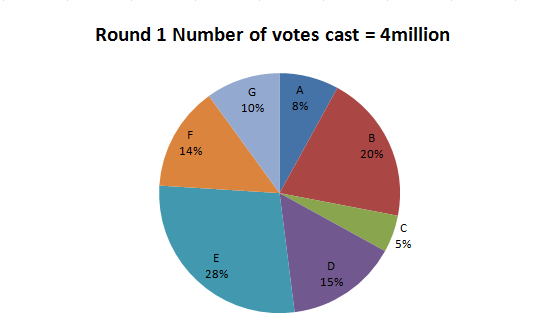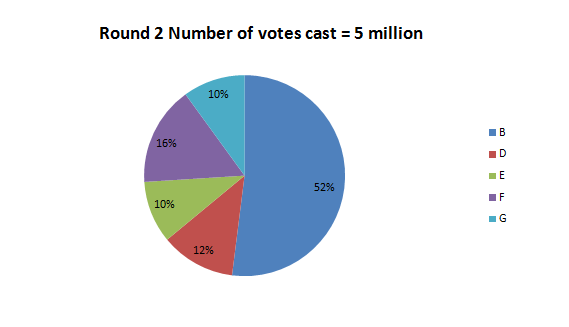Directions: Study the graphs to answer these question. In an election, if no single candidate secures a simple majority of 50% in the first round, then the top five candidates in terms of votes polled proceed to the next round. If no one still get a simple majority, then the top three of that round go into the next round. If the decision is still not decisive in favour of one candidate, only the top two proceed to the last round. The following pie charts show the results of the elections.Question 1: How many more votes were cast in favour of candidate G in Round 2 as compared to Round 1?
(a) 1 million
(b) 1 lakh
(c) 0.01 million
(d) None of these

Question 2: Which of the following candidates got equal number of votes in both the rounds?
(a) E
(b) D
(c) B
(d) G

Question 3: The law states that every candidate other than the top five candidates in Round 1 must pay Rs 20 for
every vote less than the votes of the lowest of top five. How much will the government earn through this source
(a) Rs 5.6 million
(b) Rs 6 million
(c) Rs 56 million
(d) None of these

Question 4: Ratio of the votes secured by candidate E in the first round to those by candidate B in the second round is
(a) 28 : 27
(b) 4 : 5
(c) 2 : 5
(d) 28 : 65

From the given information, we can make the following table:

 ROUND 1 %age of votes Votes in million A 8 0.32 B 20 0.8 C 5 0.2 D 15 0.6 E 28 1.12 F 14 0.56 G 10 0.4 4 million ROUND 2 %age of votes Votes in million B 52 2.6 D 12 0.6 E 10 0.5 F 16 0.8 G 10 0.5 5 million

The votes got by G in the first round = 0.4 million

and the votes got by G in the second round = 0.5 million

The required difference = 0.5-0.4= 0.1 million = 1 lakh.

The correct option is (b)

D got 6 lakh votes in both rounds.

The correct option is (b)

The two candidates at the bottom of the first round are A and C and the lowest person among top five is G who got 0.4 million votes.

Hence, amount paid by A = (0.4 – 0.32) × 20 million = Rs 1.6 million

The amount paid by C = (0.4 – 0.2) × 20 million = Rs 4 million

Hence, the government will earn = 1.6 + 4 = Rs 5.6 million

The correct option is (a)

The votes secured by candidate E in the first round 1.12 million

The votes secured by candidate B in the second round = 2.6 million

Required ratio = 1.12:2.6 =28:65

Correct option is (d)

### Want to explore more Data Interpretation Sets?

Get Posts Like This Sent to your Email
Updates for Free Live sessions and offers are sent on mail. Don't worry: we do not send too many emails..:)
Get Posts Like This Sent to your Email
Updates for Free Live sessions and offers are sent on mail. Don't worry: we do not send too many emails..:)